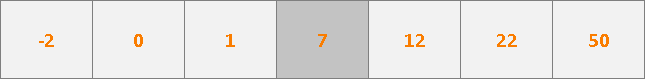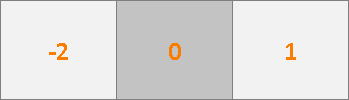Yashb | Binary Search

# Binary Search

• by Warawreh
• Last updated: 2022-01-31 11:25:26
• Estimated time to read: 10 minutes

## Binary search is a searching algorithm used to find a value in a sorted list (array) in logarithmic time (very efficient), by dividing the values in the array into two parts and by looking at the value in the middle we decide which part to keep and which part to discard, then we do the same operations on the half we kept and so on until we find the value we need.

1. Divide the values into two parts.
2. Check the value in the middle.
3. Keep one-half of the values and discard the other.
4. If there is only one value we decide to take it or not and terminate the process.
5. If there is more than one value repeat step 1.
Now let's see how much time does it take, each time we do the Checking part let's say it takes $$C(N)$$ time depending on the value given, and it is done multiple times and it can be calculated like this:

$$T(N) = C(Y) + T({N \over 2})$$, Where $$N$$ is the number of values and $$Y$$ is the value in the middle

As we see the check function will repeat logN times So the total complexity is $$O(C(N) Log_2N)$$.

## Example

We want to solve the following task: find how many values are more than or equal to X in a sorted array a.
input:
a = {-2 ,0 ,1 ,7 ,12 ,22 ,50}
x = 7
Output:
Number of values in a more than 7: 4.

Let's follow the steps of binary search, but first, we need to choose a check function that will help us to find the number of values more than or equal to x, we can easily find the smallest number more than or equal to x and find all values from it, so the check function will be :

$$C(i)$$ = True if $$a[i] \ge x$$
$$C(i)$$ = False if $$a[i] < x$$

And if $$C(i)$$ is true we take the left side (since we want to find the smallest value more than or equal to x) and discard the right side and vice versa, Note: if $$C(A)$$ is true we need to save the value of i every time $$C(i)$$ is true.7 is the middle value, [-2, 1] is the left half, [12,50] is the right half
Here i = 3 and a[i] = 7, $$C(3)$$ is True $$(7 \ge 7)$$ so we discard the right side [12,50] and keep the left side [-2,1] and save the value of i in the answer.
Note: the middle value is discarded since it is not part of the left half, also it is not needed since if it is the answer we need it is already saved inside the answer else it is discarded.0 is the middle value, [-2, -2] is the left half, [1,1] is the right half

Here i = 1 and a[i] = 0, $$C(0)$$ is False $$(0 < 7)$$ so we discard the left side [-2,-2] and keep the right side [1,1].1 is the middle value, [] is the left half, [] is the right half

Here i = 2 and a[i] = 1, $$C(1)$$ is False $$(1 < 7)$$ so we discard the left side [] and keep the right side [].
Note: i = 2 because we remember the indices of the values in the original array, not in the new array.

Now the array of values we want to work on is empty so we terminate the process and calculate the answer, the saved answer is 3, which is the index of the first value more than or equal to so the real answer is n - answer where is the number of values in the array 7 - 3 = 4.

## Implementation

#include <iostream>using namespace std;

int binarySearch(int n,int array[],int x){

//The current bounders we will work on
int lower = 0,higher = n-1;
//The index of the smallest number more than or equal to x
//-1 means there are no value more than or equal to x
int at = -1;

//Repeat while the bounders represent an array with a size of more than 0
while(lower <= higher){
//Find the middle value
int middle = (lower + higher) / 2;
//The check function start
bool good = array[middle] >= x;
//The check function end

if(good){
//We discard the right half (from middle to higher)
//We save the the left half (from lower to middle - 1)
higher = middle - 1;
//We save the current value in at
at = middle;
}else{
//We discard the left half (from lower to middle)
//We save the the left half (from middle + 1 to higher)
lower = middle + 1;
}
}
//The answer is all the values from 'at' to 'n'
int answer = n - at;
}

int main(){

int n = 7;
int values[] =  {-2 ,0 ,1 ,7 ,12 ,22 ,50};
int x = 7;

cout << "Number of values in a more than " << x << ": " << binarySearch(n,values,x) << '\n';
}
Space complicity is O(N) to save the array, Time complicity is O(LogN).